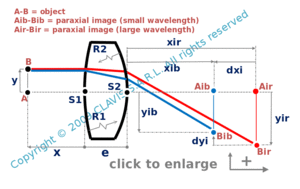and orgeometrical optics
chromatism

height chromatism of a thick lens

Caution
Fill in all input cells.
Choose all parameters unit.
ns and nl can be either values or the reference of a glass from the catalog. If a value is directly entered, the wavelength will not be considered.
Except e which is positive, distances and heights are algebric.
Radii are algebric or infinite. Enter "infinite" for a plane surface.
Positive directions are according the arrows in the bottom right of the scheme.

InputValueunit
x
y
nl
ns
R1
R2
e
OutputValueUnit
xirto be calculated
xibto be calculated
yirto be calculated
yibto be calculated
dyito be calculated
rto be calculatedx : distance from the vertex of the first surface to the object point y : object's height nl : refraction index for the large wavelength ns : refraction index for the small wavelength R1 : radius of curvature of the first surface R2 : radius of curvature of the second surface xir : distance from the vertex of the second surface to the image point for the large wavelength xib : distance from the vertex of the second surface to the image point for the small wavelength yir : image's height for the large wavelength yib : image's height for the small wavelength r : ratio dyi/ yi with yi = (yli + ysi)/2

Note
This calculation page can not detect all unrealistic inputs.